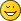# phyphox Forums

Full Version: Questions About GPS Measurements
You're currently viewing a stripped down version of our content. View the full version with proper formatting.
[attachment=558]Hello! I like to use Phyphox to record my walking and biking trips using the Location GPS app.

When I export the data from a session, a rich set of data is provided. I have a couple questions about this data:

What exactly do Horizontal Accuracy and Vertical Accuracy mean / where do these values come from?
It seems unrealistic to assume there is a guarantee that my actual position is within x meters of the measured position. My guess is the accuracy expresses an uncertainty that perhaps can be given by a distribution where, say, I can be 95% confident my actual position is within x meters of the measured position. Is that the case?

This leads to my second question:

How is Distance (km) data computed?
A simple way would be to compute the straight-line distance between two (measured) positions. However, given the uncertainty in the measurement, I think this tend to overstate the actual distance traveled in the time delta t. Is any correction applied for an expected overstatement based on the accuracy values?

Thanks for looking at my questions!
(10-08-2022, 02:19 AM)aholaday Wrote: [ -> ]What exactly do Horizontal Accuracy and Vertical Accuracy mean / where do these values come from?
It seems unrealistic to assume there is a guarantee that my actual position is within x meters of the measured position. My guess is the accuracy expresses an uncertainty that perhaps can be given by a distribution where, say, I can be 95% confident my actual position is within x meters of the measured position. Is that the case?
It is just 68% on Android, see https://developer.android.com/reference/...Accuracy(), I could not find similar information for iOS…

In addition, I have found  https://stackoverflow.com/questions/2846...confidence and keep it here just for my reference…Quote:How is Distance (km) data computed?
A simple way would be to compute the straight-line distance between two (measured) positions. However, given the uncertainty in the measurement, I think this tend to overstate the actual distance traveled in the time delta t. Is any correction applied for an expected overstatement based on the accuracy values?
We use a simple way with spherical coordinates. You could check the exact formula in https://github.com/phyphox/phyphox-exper...ps.phyphox (see phyphox file format). So, an overestimate of 5…10% would not be unexpected and I have received a report where phyphox in fact has overshot a distance in this margin.

We have briefly though about improving the algorithm, however, it is rather low priority on our list at the moment.
(10-10-2022, 01:01 PM)Jens Noritzsch Wrote: [ -> ]
(10-08-2022, 02:19 AM)aholaday Wrote: [ -> ]What exactly do Horizontal Accuracy and Vertical Accuracy mean / where do these values come from?
It seems unrealistic to assume there is a guarantee that my actual position is within x meters of the measured position. My guess is the accuracy expresses an uncertainty that perhaps can be given by a distribution where, say, I can be 95% confident my actual position is within x meters of the measured position. Is that the case?
It is just 68% on Android, see https://developer.android.com/reference/...Accuracy(), I could not find similar information for iOS…

In addition, I have found  https://stackoverflow.com/questions/2846...confidence and keep it here just for my reference…Quote:How is Distance (km) data computed?
A simple way would be to compute the straight-line distance between two (measured) positions. However, given the uncertainty in the measurement, I think this tend to overstate the actual distance traveled in the time delta t. Is any correction applied for an expected overstatement based on the accuracy values?
We use a simple way with spherical coordinates. You could check the exact formula in https://github.com/phyphox/phyphox-exper...ps.phyphox (see phyphox file format). So, an overestimate of 5…10% would not be unexpected and I have received a report where phyphox in fact has overshot a distance in this margin.

We have briefly though about improving the algorithm, however, it is rather low priority on our list at the moment.

Thanks Jens! This makes sense, and I appreciate the helpful references. I am using iOS so maybe I will have to do an experiment to determine the sphere of uncertaintyI appreciate you taking to the time to reply!
We are looking forward to your results.